+ Text Only Site
+ Non-Flash Version
+ Contact GlennDisplacement Answers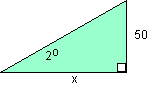A full-sized glider has a glide angle of 2 degrees. If it loses 50 meters of altitude, how far would it move horizontally? Tan 2o = 50/x Therefore, x = 50/Tan 2o = 1432 m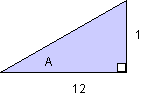A model glider moves horizontally 12 meters for every meter of altitude it loses. What is it's glide angle? Tan A = 1/12 Therefore, LA = 4.8o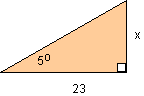A model glider has a glide angle of 5 degrees. If it flies 23 meters horizontally, how much altitude will it lose?  Tan 5o = X/23 X = 23 (Tan 5o) = 2 m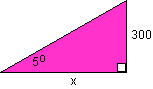A full-sized glider has a glide angle of 5 degrees. If the altitude is 300 meters, will the glider make it to an airport 4,000 meters away? Tan 5o = 300/X X= 300/Tan 5o = 3,429 m (Note: Will not make it to the airport 4,000 m away.)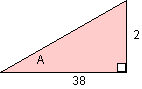A full-sized glider loses 2 meters for every 38 meters it travels horizontally. What is it's glide angle? Tan LA = 2/38 Therefore, LA = 3.0o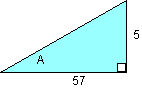A model glider travels 57 meters horizontally after losing 5 meters of altitude. What is it's glide angle? Tan LA = 5/57 Therefore, LA = 5.0o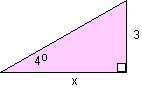A model glider loses 3 meters of altitude. If it has a glide angle of 4 degrees, how far did the glider travel horizontally? Tan 4o = 3/X X = 3/Tan 4o X = 42.9 m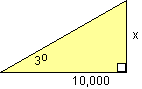A full-sized glider lands 10,000 meters horizontally from where it began its downward diagonal path. If the glide angle is 3 degrees, what was the glider's altitude? Tan 3o = X/10,000 X = (10,000)(Tan 3o) X = 524 m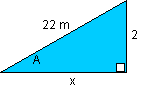A model glider has an average velocity of 2.2 m/s along it's downward diagonal path. After 10 seconds, the glider has lost 2 meters of altitude. What is the glider's glide angle and how far horizontally did it travel? d = Vt (Note: a = 0) d = (2.2)(10) = 22 m (hyp) Sin LA = 2/22 Therefore, LA = 5.2o 222 = 22 + X2 X = sq. root of (222 - 22) = 21.9 m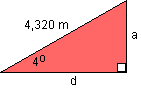A full-sized glider has a velocity of 36 m/s along it's downward diagonal path. If it has a glide angle of 4 degrees, how much altitude will it lose in 2 minutes? How far will it travel horizontally in that 2 minutes? d = Vt (Note: a = 0) d = 36 (120s) = 4,320 m Sin 4o = a/4320 a = (4320)(Sin 4) = 301 m Cos 4o = d/4320 Therefore, d = (4320)(Cos 4) = 4,309 m

Related Pages:
Standards
Activity
Worksheet
Lesson Index
Aerodynamics Index+ Inspector General Hotline + Equal Employment Opportunity Data Posted Pursuant to the No Fear Act + Budgets, Strategic Plans and Accountability Reports + Freedom of Information Act + The President's Management Agenda + NASA Privacy Statement, Disclaimer, and Accessibility CertificationEditor: Tom Benson NASA Official: Tom Benson Last Updated: Thu, May 13 02:38:26 PM EDT 2021 + Contact Glenn